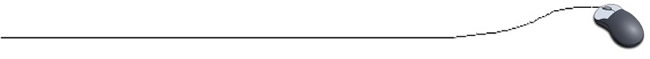Alphabetic SortingSorting into alphabetic order can be accomplished with both the bubble and exchange processes.
The only thing
you must remember when sorting alphabetically is the way in which Java deals with comparing String values.
• Java provides two methods for comparing strings: compareTo and compareToIgnoreCase

• If s1 and s2 are String variables, then their values can be compared by s1.compareTo(s2).

• compareTo returns an int which is 0 if the two strings are identical, positive if s1 > s2, and negative if s1 < s2.

• compareToIgnoreCase operates exactly as does compareTo except that it handles mixed case strings as single case strings

ASCII (Anerican Standard Code for Information Interchange) is a standard for character encoding. It represents text in computers and other devices. (ASCII code is a subset of Unicode.)

The ASCII code chart assigns numerical values to the sequences ‘a-z’, and ‘A-Z’.  For example, the capital letter A is assigned a numerical value (065) smaller than that for capital B (066). Lower case a is assigned 097 and lower case b is assigned 098.   Thus alphabetic order is actually ascending order.

//Bubble Sort for Alphabetical Order:
public class AlphaSortingBubble
{
public static void main(String[ ] args)
{
String[ ] names = {"joe", "slim", "ed", "george"};
sortStringBubble (names);
for ( int k = 0;  k < 4;  k++ )
System.out.println( names [ k ] );
}
//Method for Bubble Sort
public static void sortStringBubble( String  x [ ] )
{
int j;
boolean flag = true;
// will determine when the sort is finished
String temp;

while ( flag )
{
flag = false;
for ( j = 0;  j < x.length - 1;  j++ )
{
if ( x [ j ].compareToIgnoreCase( x [ j+1 ] ) > 0 )
{                                             // ascending sort
temp = x [ j ];
x [ j ] = x [ j+1];     // swapping
x [ j+1] = temp;
flag = true;
}
}
}
}
}

//Exchange Sort for Alphabetical Order:
public class AlphaSortingExchange
{
public static void main(String[ ] args)
{
String[ ] names = {"joe", "slim", "ed", "george"};
sortStringExchange (names);
for ( int k = 0;  k < 4;  k++ )
System.out.println( names [ k ] );
}
//Method for Exchange Sort
public static void sortStringExchange( String  x [ ] )
{
int i, j;
String temp;

for ( i = 0;  i < x.length - 1;  i++ )
{
for ( j = i + 1;  j < x.length;  j++ )
{
if ( x [ i ].compareToIgnoreCase( x [ j ] ) > 0 )
{                                             // ascending sort
temp = x [ i ];
x [ i ] = x [ j ];    // swapping
x [ j ] = temp;

}
}
}
}
}